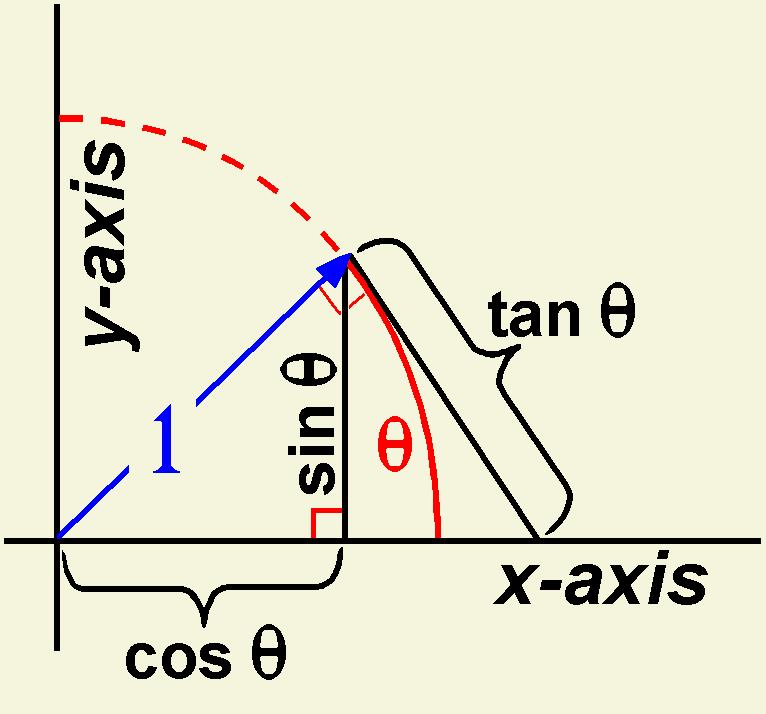# How do you verify (cos(x))(cos(x)) = 1-sin^2(x)?

Nov 12, 2015

We can rewrite the equation as ${\cos}^{2} \left(x\right) = 1 - {\sin}^{2} \left(x\right)$, and thus as

${\cos}^{2} \left(x\right) + {\sin}^{2} \left(x\right) = 1$

This is a fundamental equality for the trigonometric function, and its explanation is very simple: if you consider the unit circle, any point on the circumference $\left(x , y\right)$ is of the form (cos(alpha), sin(alpha), for some angle $\alpha$ which identifies the point.

Since $\cos \left(\alpha\right)$ is the $x$ coordinate and $\sin \left(\alpha\right)$ is the $y$ coordinate of the point, we have that $\cos \left(\alpha\right)$ and $\sin \left(\alpha\right)$ are the catheti of a right triangle, whose hypotenusa is the radius, which is one. So, we have that

${\cos}^{2} \left(\alpha\right) + {\sin}^{2} \left(\alpha\right) = {1}^{2} = 1$

I hope this image can help, since it shows that the sine and cosine of an angle form a right triangle whose hypotenusa is the radius.